# Polynomial Functions

Polynomial Functions
Go back to  'Functions'

All of us have been studying polynomials since we were quite young. When we were first told about variables and expressions, we were simply dealing with polynomials.

This is a branch of math where you can relax because polynomials are relatively easy to learn.In this mini-lesson, we will explore the world of polynomial functions in math. You will get to learn about the highest degree of the polynomial, graphing polynomial functions, range and domain of polynomial functions, and other interesting facts around the topic. You can also check out the playful calculators to know more about the lesson and try your hand at solving a few interesting practice questions at the end of the page.

## What Is a Polynomial Function?

### Polynomial Function Definition

A function $$f: \mathbb{R} \rightarrow \mathbb{R}$$ defined as $$f(x)=a_{n}x^{n}+a_{n-1}x^{n-1}+...+a_{2}x^2+a_{1}x+a_0$$ is called a polynomial function in variable $$x$$.

Here, $$a_0,a_1,...,a_n$$ are real number constants and $$n$$ is a non-negative integer.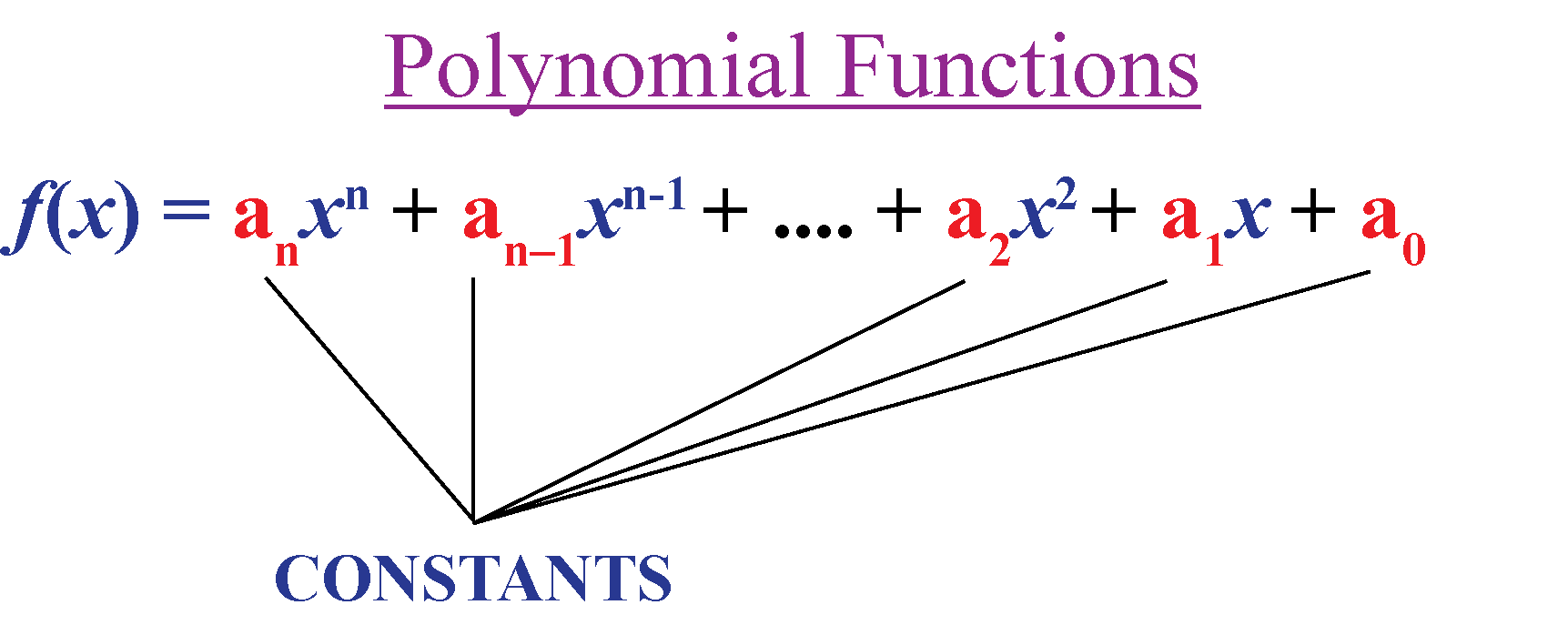If the constant $$a_n$$ is non-zero, we say this is a polynomial function of degree $$n$$ and $$a_n$$ is the leading coefficient.

### Examples

Look at the following examples.

Functions Polynomial Function or Not?
1. $$f(x)=8x^2+7x-1$$ Yes
2. $$f(x)=4x^2-9$$ Yes
3. $$f(x)=6x+8$$ Yes
4. $$f(x)=x^{\frac{2}{3}}+2x$$ No

Look at Example 4

Here, the power of $$x$$ is $$\dfrac{2}{3}$$ which is not a non-negative integer. Therefore, it is not a polynomial function.

The remaining functions are polynomial functions.

### Degree of the Polynomial Function

The degree of the polynomial function is determined by the highest power a variable it is raised to,

For example, the degree of $$-x^4+x^2+x$$ is 4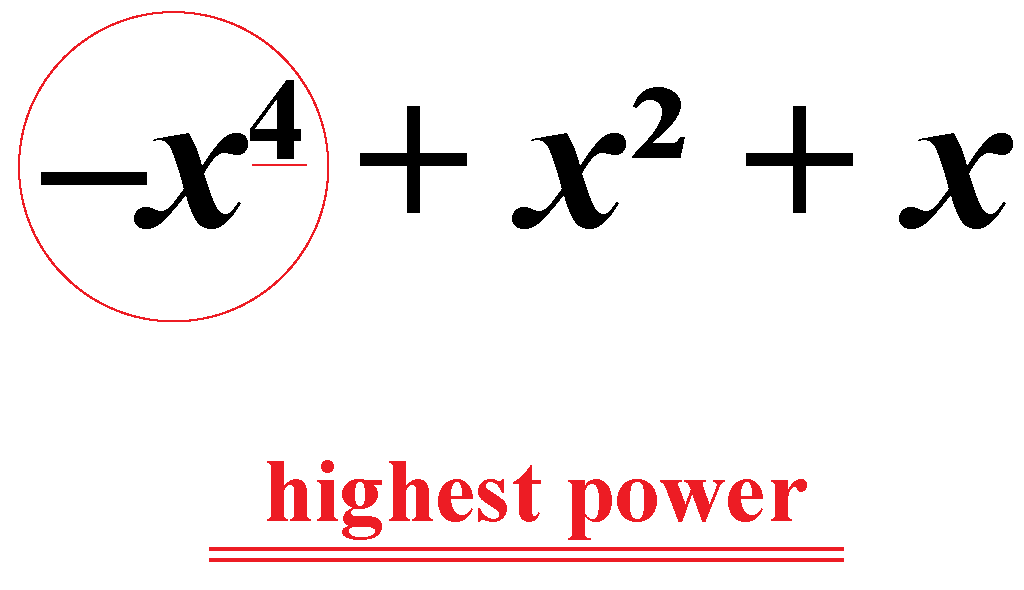## What Are the Types of Polynomial Functions?

The 5 types of polynomial functions are:

1. Zero Polynomial Function
2. Linear Polynomial Function
4. Cubic Polynomial Function
5. Quartic Polynomial Function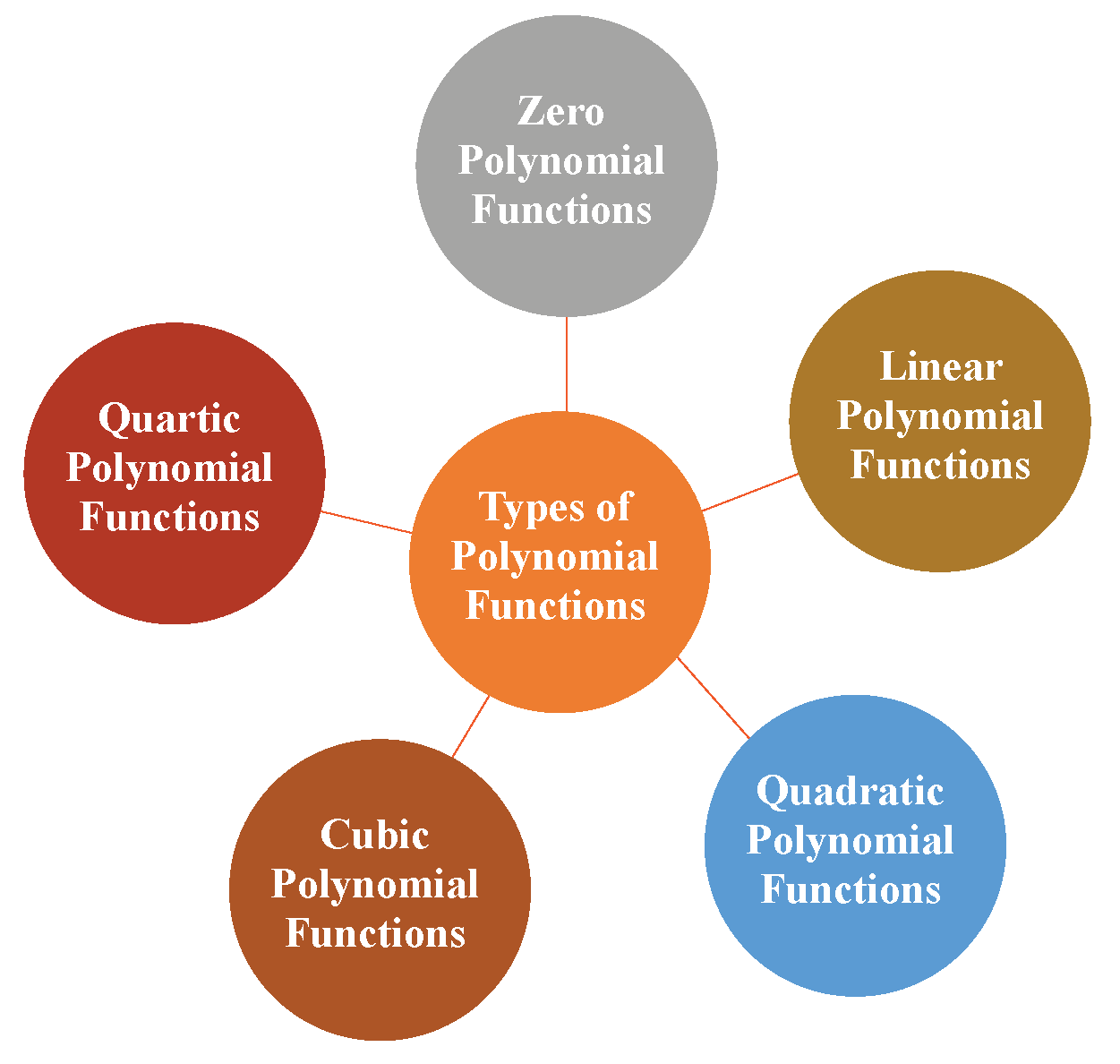## How Do You Determine a Polynomial Function?

If a function $$f: \mathbb{R} \rightarrow \mathbb{R}$$ is defined as $$f(x)=a_{n}x^{n}+a_{n-1}x^{n-1}+...+a_{2}x^2+a_{1}x+a_0$$, then we say that the function is a polynomial function.

Remember a few points while determining if a function is a polynomial function or not.

1. If the function is in variable $$x$$, make sure all the powers of $$x$$ is a non-negative integer. For example, $$f(x)=\dfrac{1}{x^2}$$ is not a polynomial function.
2. The function should not contain any square roots or cube roots of $$x$$.
3. Sometimes, a polynomial function is NOT written in its standard form. For example, although the function $$f(x)(x-1)(x+2)$$ is not written in the standard form, it is a polynomial function.
4. The variable should not be in the denominator.

Here are a few examples of polynomial functions.

Polynomial Functions Examples
1. Linear Polynomial Function $$f(x)=5x-9$$
2. Quadratic Polynomial Function $$f(x)+x^3=x(x^2+x-3)$$
3. Cubic Polynomial Function $$f(x)-x^3=-3x+1$$

## How to Represent a Polynomial Function on Graph?

### Graphing Polynomial Functions

Let the polynomial function be $$y=f(x)$$.

Draw a table for $$y$$ and $$f(x)$$ values to draw a graph of the polynomial function.

Mark the points on x-axis and y-axis and plot the points obtained in the table.

Join the points to obtain the curve.

Here are a few graphs of polynomial functions.

### Linear Polynomial Functions

If $$f(x)$$ is a constant, then the graph of the function forms a vertical line parallel to the y-axis and vice-versa.

The graph of a linear polynomial function always forms a straight line.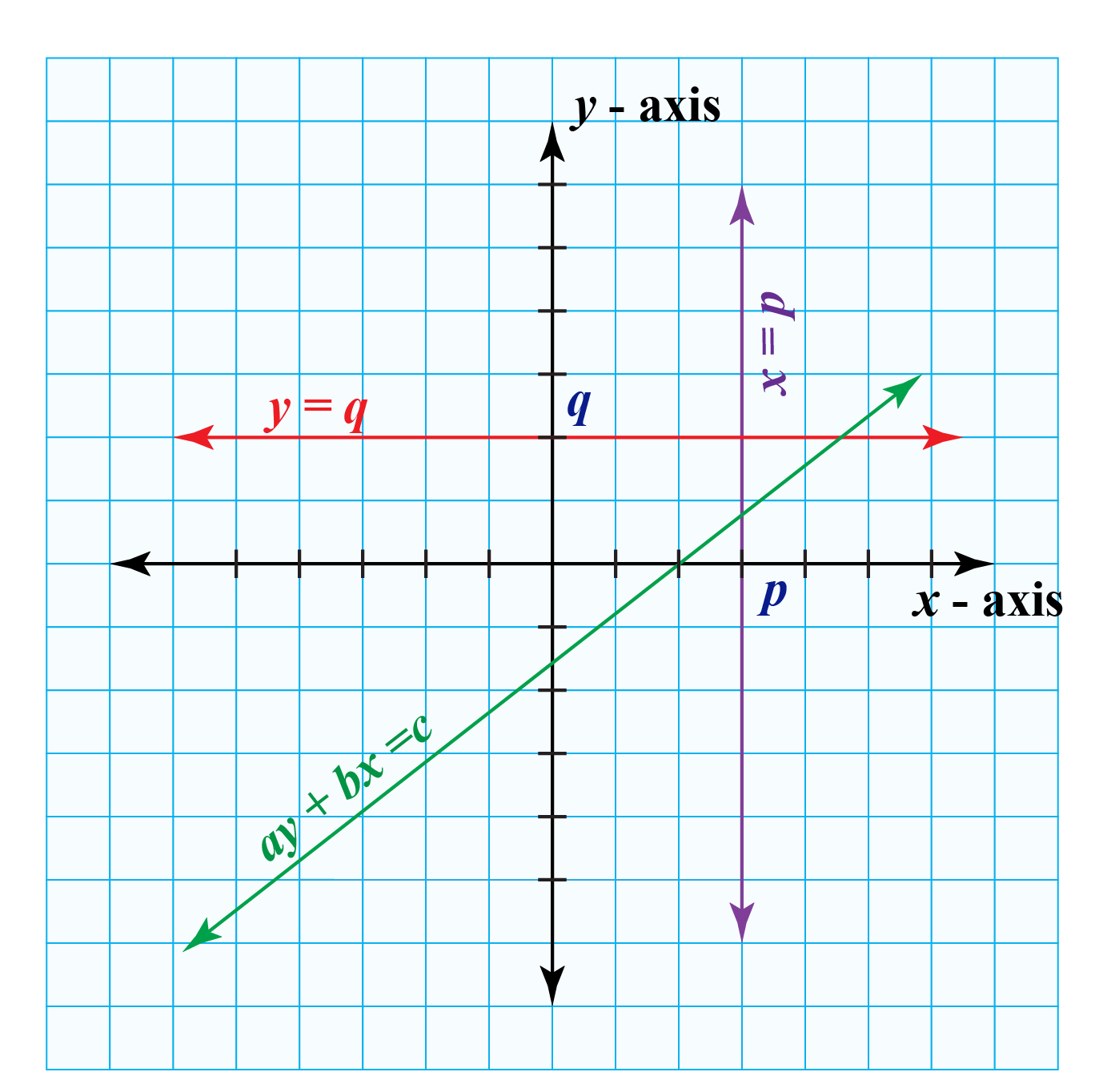The reason a polynomial function of degree one is called a linear polynomial function is that its geometrical representation is a straight line.

This is how the quadratic polynomial function is represented on a graph.

This curve is called a parabola.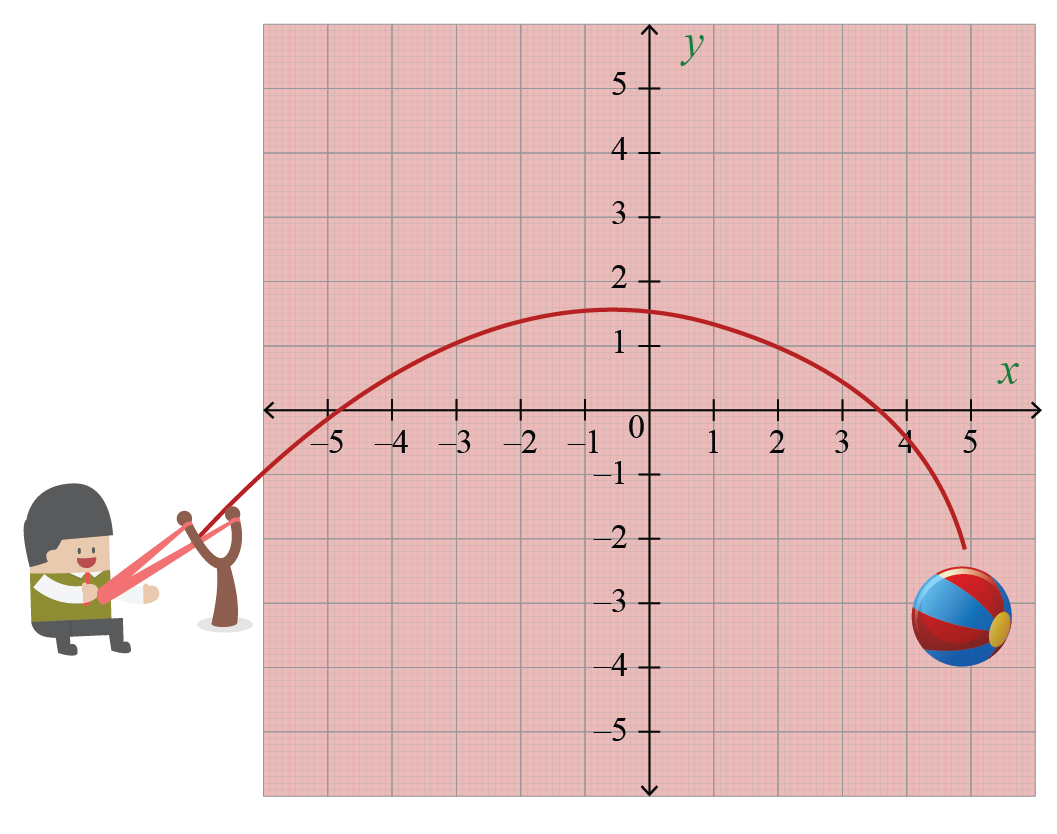Here is the graph of the quadratic polynomial function $$f(x)=2x^2+x-3$$### Cubic Polynomial Functions

Look at the shape of a few cubic polynomial functions.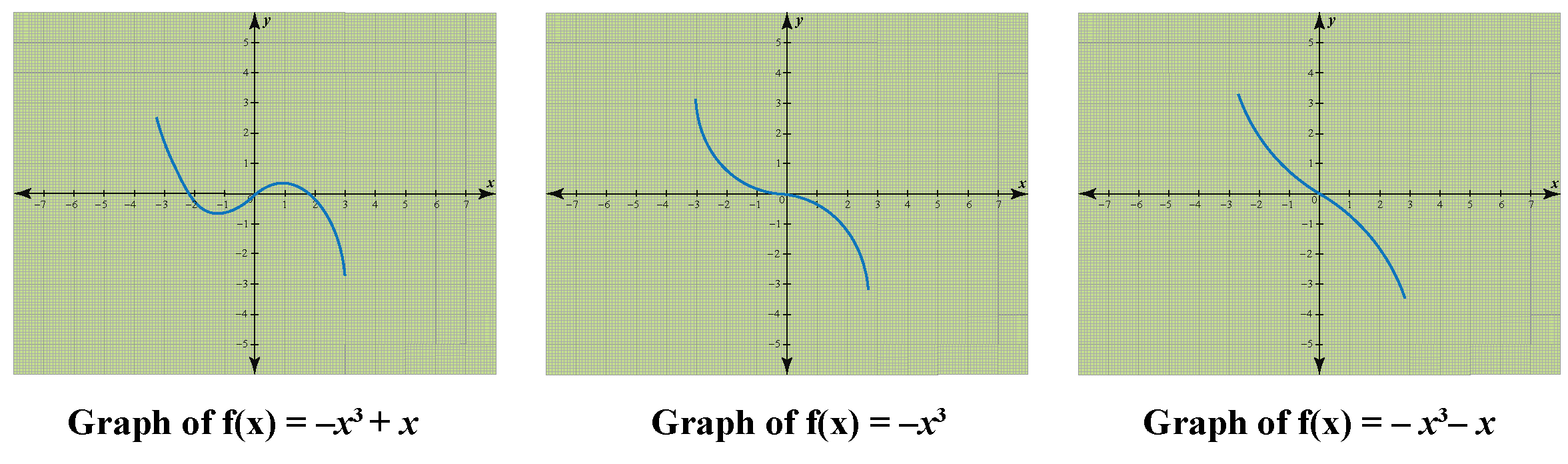Did you notice that the curves are symmetric about a point under $$180^{\circ}$$ rotation?

Observe that the graphs of all the polynomial functions are everywhere continuous and defined.

So, the domain of a polynomial function is $$\mathbb{R}$$.

All the values of the polynomial function lie in the set of real numbers.

So, the range of a polynomial function is also $$\mathbb{R}$$.

## Solved Examples

 Example 1

Jack shows a function to his friends in his school.He asked them to determine if it is a polynomial function?

Can you help them?

Solution

The function is $$f(x)=12x-5x(x+3)$$

Expand the bracket on the right side and simplify.

\begin{align}f(x)&=12x-5x(x+3)\\&=12x-5x^2-15x\\&=-5x^2-3x\end{align}

 Yes, the function is a polynomial function.
 Example 2

Mia is a fitness enthusiast who goes running every morning.

The park where she jogs is rectangular in shape and measures 12 feet by 8 feet.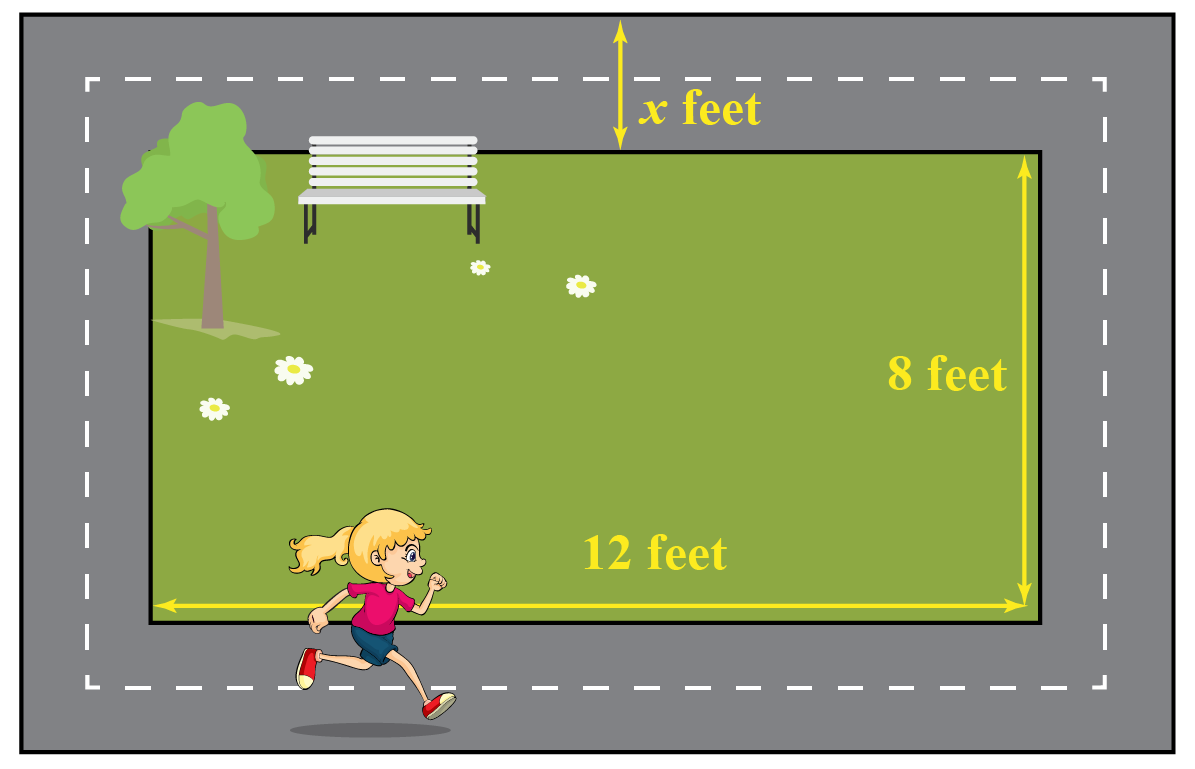A nature restoration group plans to revamp the park and decides to build a pathway surrounding the park.

This would increase the total area to 140 sq. ft.

Can you use this information to establish a quadratic polynomial function?

Solution

Let’s denote the width of the pathway as $$x$$.

Then, the length and breadth of the outer rectangle is $$(12+2x)\;\text{ft.}$$ and $$(8+2x)\;\text{ft.}$$

The area of the park,
\begin{align}(12+2x)(8+2x)&=140\\2(6+x)\cdot 2(4+x)&=140\x+6)(x+4)&=35\\x^2+10x-11&=0\end{align} The quadratic function \(f(x)=x^2+10x-11

 $$\therefore$$, The required quadratic polynomial function is $$f(x)=x^2+10x-11$$.
 Example 3

We define a polynomial function $$f: \mathbb{R} \rightarrow \mathbb{R}$$ as $$f(x)=x^2$$.

Complete the table shown below.

$$x$$ -4 -3 -2 -1 0 1 2 3 4
$$f(x)=x^2$$

Find the domain and range of the function.

Solution

Let's complete the given table by finding the values of the function at the given values $$x$$.

$$x$$ -4 -3 -2 -1 0 1 2 3 4
$$f(x)=x^2$$ 16 9 4 1 0 1 4 9 16

Let's draw the graph of the function.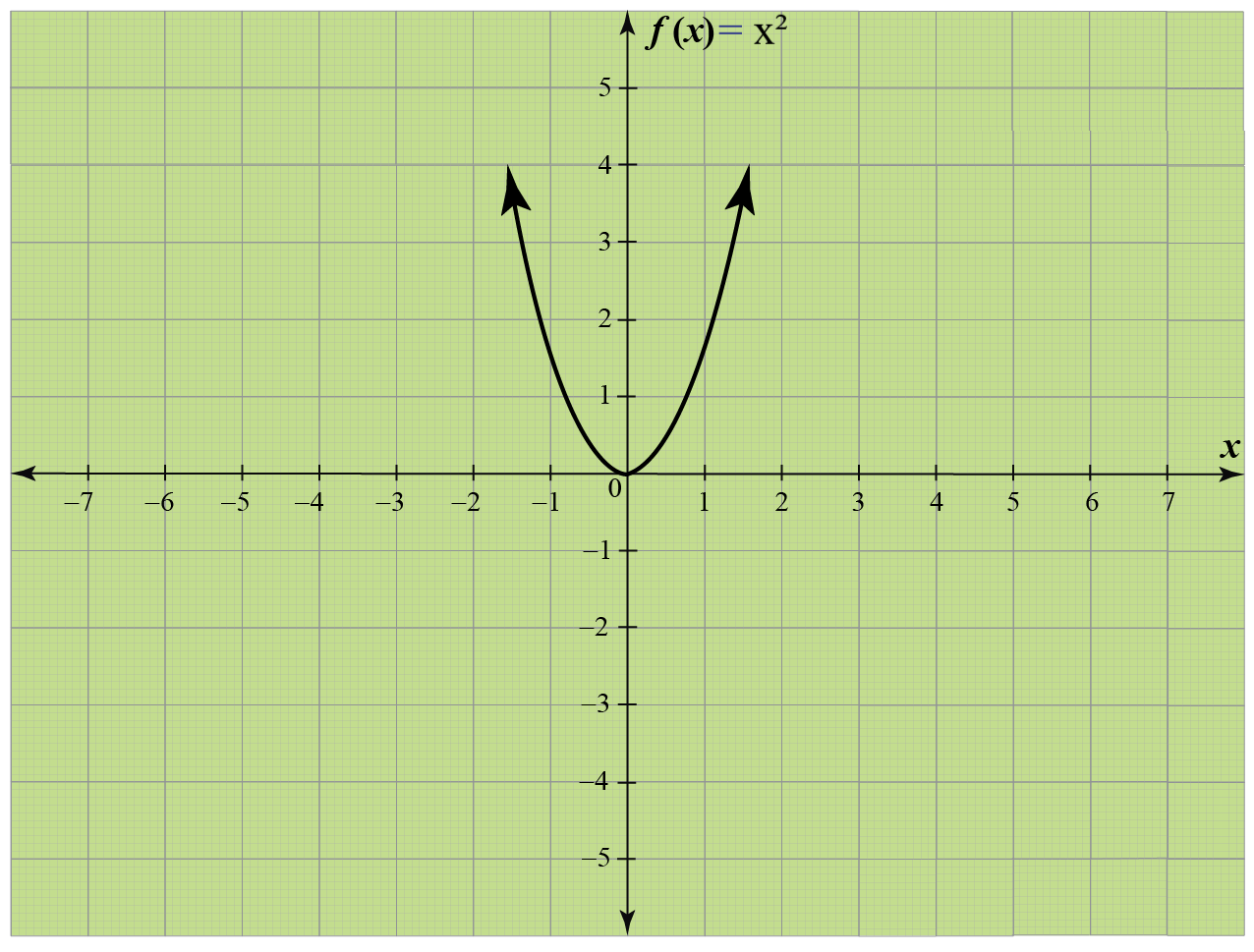So, the graph of $$f(x)=x^2$$ is shown.Think Tank
1. The sum of two numbers is 45. After subtracting 5 from both numbers, the product of the numbers is 124. What are the numbers?
2. Which of the following graphs may represent the equation $${y+2= -2(x-1)}$$?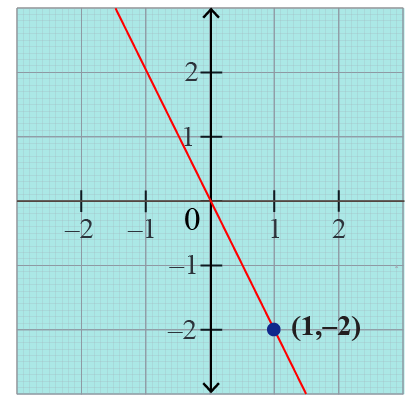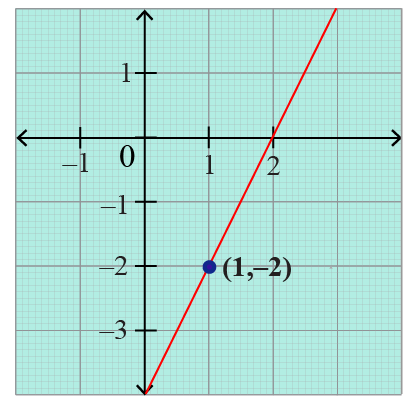## Interactive Questions

Here are a few activities for you to practice.

## Let's Summarize

This mini-lesson targeted the fascinating concept of Polynomial Functions. The math journey around Polynomial Functions starts with what a student already knows, and goes on to creatively crafting a fresh concept in the young minds. Done in a way that not only it is relatable and easy to grasp, but also will stay with them forever. Here lies the magic with Cuemath.

We hope you enjoyed learning about graphing polynomial functions, the degree of the polynomial functions, range and domain of the polynomial function in this lesson.

At Cuemath, our team of math experts is dedicated to making learning fun for our favorite readers, the students!

Through an interactive and engaging learning-teaching-learning approach, the teachers explore all angles of a topic.

Be it worksheets, online classes, doubt sessions, or any other form of relation, it’s the logical thinking and smart learning approach that we at Cuemath believe in.

### 1. How do you tell if an equation is a polynomial function?

An equation of the form $$f(x)=a_{n}x^{n}+a_{n-1}x^{n-1}+...+a_{2}x^2+a_{1}x+a_0$$, where $$a_0,a_1,...,a_n$$ are constants and $$n$$ is a non=negative integer is called a polynomial function.

### 2. What are not polynomial functions?

If a function is not in the form $$f(x)=a_{n}x^{n}+a_{n-1}x^{n-1}+...+a_{2}x^2+a_{1}x+a_0$$, then it is not a polynomial function. For example, $$f(x)=\cos{x}$$.

### 3.  Is 0 a polynomial function?

Yes, all constants are polynomial functions. So, 0 is a polynomial function.

### 4. What are the types of polynomial functions?

The 5 types of polynomial functions are:

1. Zero Polynomial Function
2. Linear Polynomial Function
4. Cubic Polynomial Function
5. Quartic Polynomial Function

### 5. What is the order of a polynomial function?

The order of a polynomial function is the same as the degree of the polynomial function.

### 6. What is an example of a polynomial function?

An example of a polynomial function is $$f(x)=x^2+3x-9$$.

### 7. What are not polynomials examples?

If a expression is not in the form $$p(x)=a_{n}x^{n}+a_{n-1}x^{n-1}+...+a_{2}x^2+a_{1}x+a_0$$, then it is not a polynomial. For example, $$f(x)=\cos{x}$$, $$f(x)=x^{\frac{2}{3}}+2x$$

### 8. What is a degree polynomial function?

A function of the form $$f(x)=a_{n}x^{n}+a_{n-1}x^{n-1}+...+a_{2}x^2+a_{1}x+a_0$$, where $$a_0,a_1,...,a_n$$ are constants and $$n$$ is a non=negative integer is called a polynomial function.

If the constant $$a_n$$ is non-zero, we say this is a polynomial function of degree $$n$$.

### 9. How do you write a polynomial function?

A function of the form $$f(x)=a_{n}x^{n}+a_{n-1}x^{n-1}+...+a_{2}x^2+a_{1}x+a_0$$, where $$a_0,a_1,...,a_n$$ are constants and $$n$$ is a non=negative integer is called a polynomial function.

Functions
Functions
grade 10 | Questions Set 2
Functions